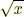index: click on a letter A B C D E F G H I J K L M N O P Q R S T U V W X Y Z A to Z index index: subject areas numbers & symbols sets, logic, proofs geometry algebra trigonometry advanced algebra & pre-calculus calculus advanced topics probability & statistics real world applications multimedia entrieswww.mathwords.com about mathwords website feedback

 Argument of a Function The variable, term or expression on which a function operates. For example, the argument ofis x, the argument of sin(2A) is 2A, and the argument of e x – 5 is x – 5. The argument of f(x) is x.     See also Sine, e